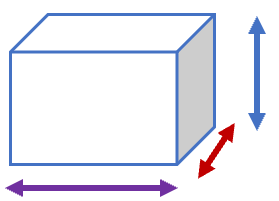# Math Worksheets Land

Math Worksheets For All Ages

# Math Worksheets Land

Math Worksheets For All Ages

# Volume of a Cube Worksheets

A cube is a peculiar shape. It is a six-sided object that all have a uniform size. The dimensions of each side are equal which makes it act as a nice measurement tool because it is a standard. You may hear of something called a unit cube. Unit cubes has a consistent capacity or volume of 1 unit which makes it a great tool to use to measure things around it. The concept of volume whether it be in a solid or liquid form is unique to students because until now they were only focused on two dimensions. Now we stepo it up to a third dimension. This selection of worksheets and lessons helps students learn how to calculate solid and liquid volume in cubic units.

### Aligned Standard: Grade 5 Measurement and Data - 5.MD.4

• Answer Keys - These are for all the unlocked materials above.

### Homework Sheets

Remember that whatever you do to the measures (find products), you must also do to their units of measure.

• Homework 1 - What is the volume of this object?
• Homework 2 - Volume of a rectangular prism = length x width x height
• Homework 3 - Use the concept presented to you to answ this best.

### Practice Worksheets

What a really neat way to go about learning this skill!

• Practice 1 - Use the single unit to fly through the problem.
• Practice 2 - Two greens and a single red to solve it.
• Practice 3 - These objects look much larger than the rest.

### Math Skill Quizzes

I see a lot of units listed as squares from students on this. Remind them that cubes get cubed.

• Quiz 1 - Some of these objects appear larger than they are.
• Quiz 2 - Lots of height presented here.
• Quiz 3 - See what you feel you know with this quiz.

### How to Measure the Volume of Cube

Cubes are six-sided shaped objects that take the form of die. You may have also heard of a heard of a cubed number which is a value that has been multiplied by itself twice. There are many different objects in the real world that have a cube shape. Sugar comes in cubes and so does ice. Milk crates are not that common any more for milk, but they are often used to store other things and also have a cubed shape. Just about any shipping company has tons of boxes moving throughout the organization in this shape. They are also interested in determining how much space those boxes take up in their trucks and planes.

By definition, volume is the measurement of the space occupied by an object. In other words, if have you a box placed on your floor, the space it occupied is called the volume of the box. There are different ways to calculate the volume of different shapes but let us consider an easier example. If you want to calculate the volume of the box on your floor, you will need to multiply the three dimensions shown in the figure. These measures include the length, height, and width. Length is the longest side of the box. Height is the vertical dimension of the box which normally faces upwards. Width is the measure from front to back.For a perfect cube, the length, width, and height are equal. Therefore, we can multiply all three of them and get the volume. Now, when you write the unit of the volume, you must see that all of these three parameters need to have the same individual unit. So, if your length, width, and height are in cm, then, by multiplying, the resultant unit becomes cm3. Let’s take out the volume whose length, width and cube are 5 cm each. The answer then becomes 5 x 5 x 5 = 125 cm3.

Unlock all the answers, worksheets, homework, tests and more!
Save Tons of Time! Make My Life Easier Now

## Thanks and Don't Forget To Tell Your Friends!

I would appreciate everyone letting me know if you find any errors. I'm getting a little older these days and my eyes are going. Please contact me, to let me know. I'll fix it ASAP.